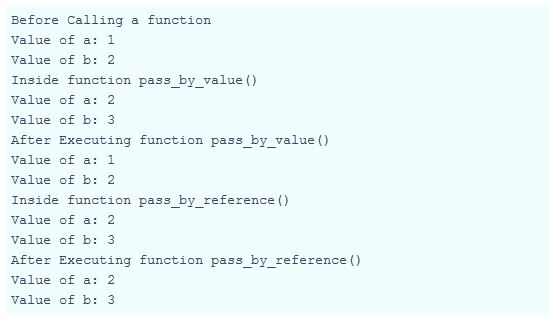# Pass by value and Pass by reference in php with example

In Pass by value the argument values are affected only locally with in the function where as in Pass by reference the argument values affected globally (i.e outside the function).

Program:` `

```\$a=1;
\$b=2;
print "Before Calling a function";
print "Value of a: " . \$a ;
print "Value of b: " . \$b ;

function pass_by_value(\$a,\$b){
\$a++;
\$b++;
print "Inside function pass_by_value()";
print "Value of a: " . \$a;
print "Value of b: " . \$b;
}

pass_by_value(\$a,\$b);
print "After Executing function pass_by_value()";
print "Value of a: " . \$a ;
print "Value of b: " . \$b;

function pass_by_ref(&\$a,&\$b){
\$a++;
\$b++;
print "Inside function pass_by_reference()";
print "Value of a: " . \$a;
print "Value of b: " . \$b;
}

pass_by_ref(\$a,\$b);
print "After Executing function pass_by_reference()";
print "Value of a: " . \$a ;
print "Value of b: " . \$b;```

Explanation:

Declare a two Variables say that \$a=1 and \$b=2

Pass by Value:

Inside the function -> passbyvalue() the argument passes the value to the function.  it will increment both value to +1 (because ++ denotes +1). After excuting function -> passbyvalue() has same value of variable \$a and \$b, since those incremented value has its scope( {-Scope-} ) with in the function.

Pass by Reference:

This is similar to the pass by value, but after executing function -> passbyreference() value of the variable will access the memory location of its reference. And returns the current memory address value (i.e the change in variable \$a and \$b with in the function will be affected globally).

Output:Category: